# simply supported beam with variable distributed load

In this tutorial we will:

• investigate shearing forces and bending moments for a simply supported beam with a variable distributed load w = f(x)
• establish general relationships between V, M and w

In the tutorials for a simply supported beam with point loading and a cantilever beam with uniform distributed load we developed the shearing force and bending moment diagrams by calculating V and M at specific points x along the beam from first principles using free body diagrams.

In this example we express V and M as mathematical functions of x from which we can obtain V and M at any point x along the beam.

Consider this example of a simply supported beam 12 m long with variable distributed load w.  The load is a linear distribution from w = 12 kN/m at x = 0 to w = 0 at x = 12.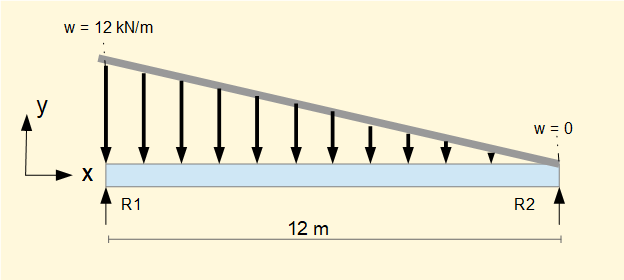The first step is to express w as a function of x, in this case a linear function with slope = -1 and intercept on the y axis = 12.

This is equivalent to a linear function     w = (12 - x)

Next step is to find the equivalent total load and its point of application.  We derive the equivalent total load through the following integral.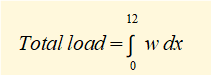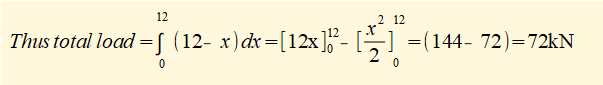This load acts at a point through the centroid of the load triangle which is 1/3 from the base at the LH end (a centroid position can also be calculated for non-linear load functions).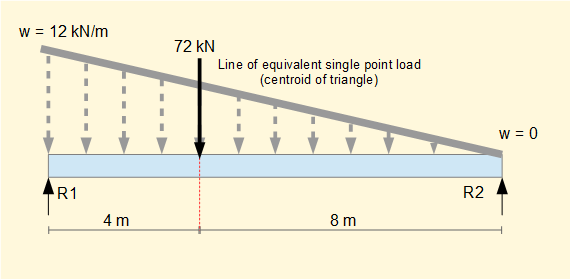Reactions R1 and R2 are then determined using Σ forces = 0 and Σ moments = 0.

Σ forces = 0 gives     R1 + R2 = 72 --------- (i)

Σ moments = 0 at x = 0 gives

(72 x 4) - (R2 x 12 ) = 0    Gives     R2 = 24 kN

Substitute R2 in (i)     gives R1 = 48 kN

Next we determine the function for the shearing force V(x) from the indefinite integral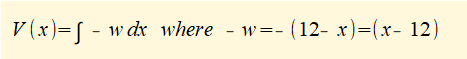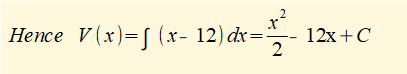To find the constant of integration C consider V(x)  at  x = 0

Substituting x = 0 in V(x) gives   V(0) = C

We know V(0) = R1 = 48 kN

Thus C = 48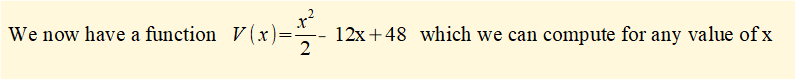Calculated values of V and the corresponding shearing force diagram are shown below. The diagram plots V at x = 0, x = 2, x = 4, x = 6, x = 8, x = 10 and x = 12 .  The true curve is a continuous quadratic function.

 x (m) 0 1 2 3 4 5 6 7 8 9 10 11 12 V (kN) 48 36.5 26 16.5 8 0.5 -6 -11.5 -16 -19.5 -22 -23.5 -24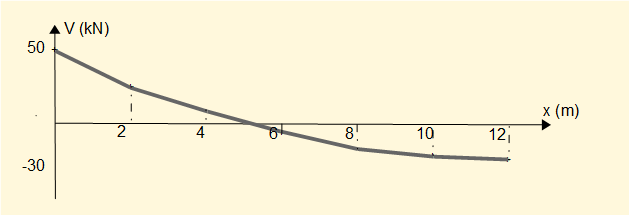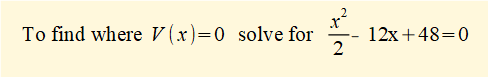for which the solution is x = 5.06

To obtain a function M(x) for the bending moment we use the relationship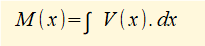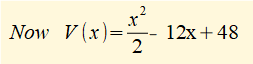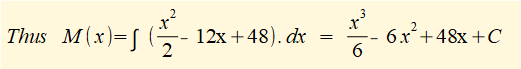To find the constant of integration C consider M(x)  at  x = 0

Substituting x = 0 in M(x) gives   M(0) = C

We know M(0) = 0    Thus C = 0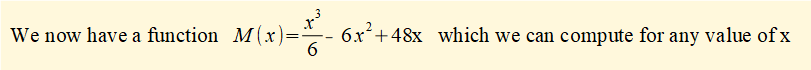Calculated values of M (rounded to integer values) and the corresponding bending moment diagram are shown below.  The diagram plots V for integer values of x in the table.  The true curve is a third order function of x.

 x (m) 0 1 2 3 4 5 6 7 8 9 10 11 12 M (kNm) 0 42 73 95 107 111 108 99 86 68 47 24 0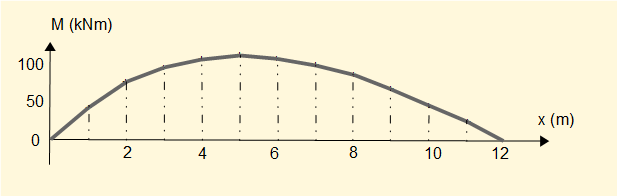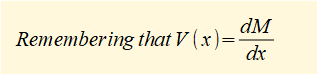it follows that the maximum value of M(x) occurs where V(x) = 0     (x = 5.06)

I welcome feedback at:

### Tutorials - mechanical vibrations

###### Forced vibrations with damping

Alistair's tutorials 2019